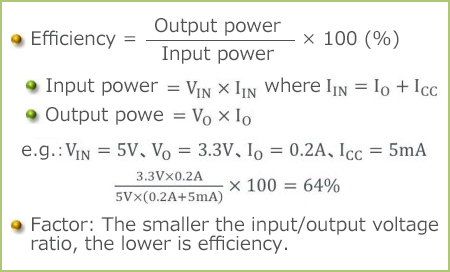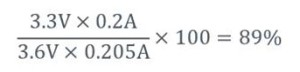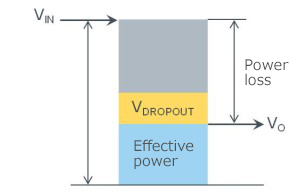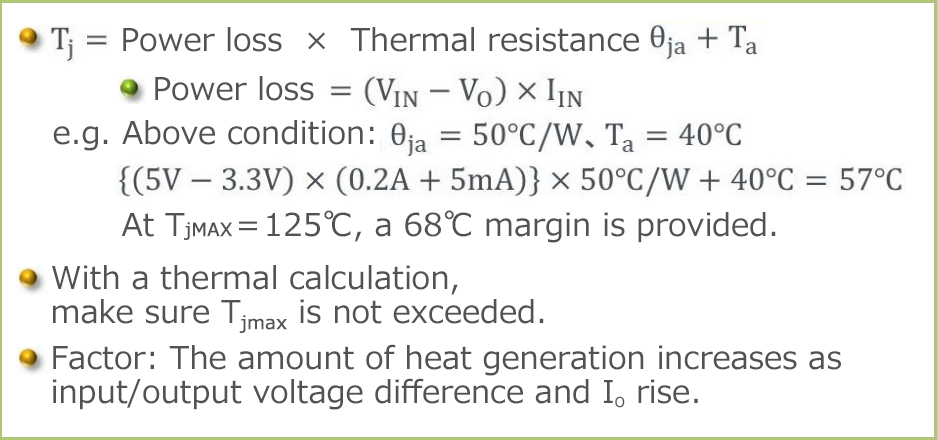# Efficiency and Thermal Calculation

2015.09.03

・An important evaluation item to prevent the heat dissipation from exceeding the TjMAX (maximum rating).

・Adjustments require a tradeoff between Ta and heat generation (power loss x thermal resistance).

This section describes the efficiency of linear regulators and thermal calculation for them. As noted above, these represent essential evaluation items in the use of a linear regulator.

## Efficiency of a linear regulatorFigure 24

The efficiency, normally indicated in %, is defined as the ratio of the output power with respect to the input power. The same definition applies to switching regulators as well. In the equation given below, the Icc contained in the input current IIN corresponds to the current consumption of the IC itself. Since this value is small, it can be ignored when the load current is large. If this is the case, the input and output currents can be treated as being equal, and the efficiency can be calculated by simply dividing the output voltage by the input voltage.

In the example illustrated in Figure 24, the efficiency of conversion from 5V to 3.3V is 64%. Given the fact that recent switching regulators have an efficiency of 80% to 90% or better, the figure of 64% seems to be low.

Let us now change the input voltage of 5V used as an example in Figure 24 to 3.6V, where 5V can be a system voltage, and 3.6V, the voltage of a rechargeable lithium ion battery.Figure 25. Power loss

Surprisingly, under this condition the efficiency is 89%. In other words, the linear regulator offers increased efficiency when the voltage difference between input and output is small, yielding a high efficiency comparable to the switching regulator. As a close examination of Figure 25 may reveal, when VIN approaches the dropout voltage VDROPOUT, the power loss declines and the efficiency increases.

Under these conditions, the LDO makes significant contribution. Because the input/output difference is 0.3V in these conditions, the linear regulator that should be selected is the LDO type, which is an LDO having a dropout voltage less than 0.3V. Standard linear regulators are unable to meet these conditions. If a standard linear regulator must be used, an input voltage greater than 6.3V is required (assuming a 3V dropout voltage), which fails to meet the initial 5V input condition. Also, the efficiency declines to 52%. Conversely, in situations where 5V must be produced from 12V, the efficiency and loss factors remain the same for both the LCO and standard type.

The efficiency of linear regulators depends on the input/output voltage difference. Although the dropout voltage is relevant to the extent to which the input/output voltage difference can be reduced, it is not directly related to efficiency. This is evident from the fact that the equation of the efficiency does not contain any dropout term. This point should not be confused.

## Key Points:

・”Efficiency” means power conversion efficiency. The same concept applies to both the linear and switching regulators.

・Under suitable conditions, linear regulators can attain the same efficiency as switching regulators.

・A key point in improving the efficiency of a linear regulator involves the question of how to reduce the power loss component depicted in Figure 25.

## Thermal calculation for a linear regulator

Thermal calculation requires information of power loss, package thermal resistance, and ambient temperature. Power loss is calculated in the same manner as the efficiency calculation: simply multiply the input/output voltage difference by the input current. Thermal resistance is provided in most data sheets. If it cannot be found, consult the manufacturer of the linear regulator. The thermal calculation basically employs the thermal resistance θja, between the chip (junction) and ambient. Some ICs provide thermal resistance θjc between junction and case. At any rate, a thermal resistance up to θja must be determined. Finally, the ambient temperature can be an assumed temperature, such as 50℃, derived from ratings for the device in which the linear regulator is to be deployed. Under severe conditions, the ambient temperature must be obtained by direct measurement.Figure 26

In one approach, determine the amount of heat generation from the IC chip based upon the power loss and thermal resistance, to which the ambient temperature is added. The result is the temperature of the chip. Verify that the calculated Tj （chip temperature） does not exceed Tjmax （maximum rating for the chip temperature）. If Tjmax is exceeded, modify any of the conditions. This is what is meant by the statement, provided in the preceding section, that not everything can be used according to specifications; rather, it is subject to restrictions by input/output voltages, output current, and ambient temperature.

As a general rule, there are few cases in which the input or output voltage can be modified in response to rating exceedance. As a countermeasure, it may be possible to reduce the load current (the output current). In such a case, the device that receives the supply of power should have as low current consumption as possible. In another approach, it may be possible to reduce the ambient temperature, such as by changing from cooling by natural convection to cooling by a fan. Or if a fan is already in use, increase its cooling capacity or review the pattern of air convection. Although attaching a heat sink to the linear regulator to reduce its thermal resistance and cut its heat dissipation may be considered, the cost and size of a heat sink could represent significant evaluation items. Also, from the point of view of reducing the heat generation by increasing the efficiency of power supply, the use of a switching regulator, to be covered in the next section, must be considered.